You are currently offline. Some features of the site may not work correctly.

# Submodular set function

Known as: Submodular, Submodular function
In mathematics, a submodular set function (also known as a submodular function) is a set function whose value, informally, has the property that the… Expand
Wikipedia

## Papers overview

Semantic Scholar uses AI to extract papers important to this topic.
Review
2014
Review
2014
• Tractability
• 2014
• Corpus ID: 6107490
Submodularity is a property of set functions with deep theoretical consequences and far– reaching applications. At first glance… Expand
•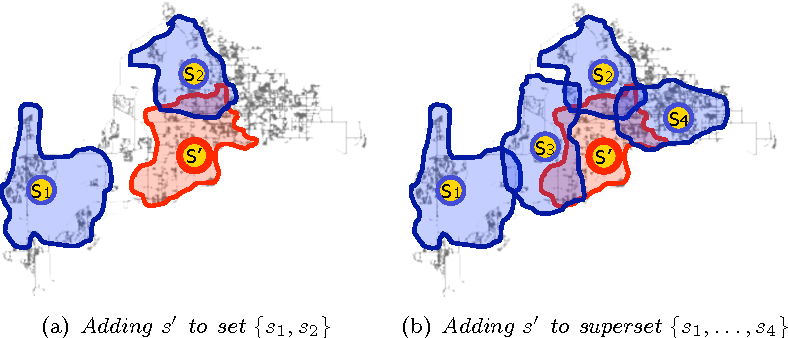•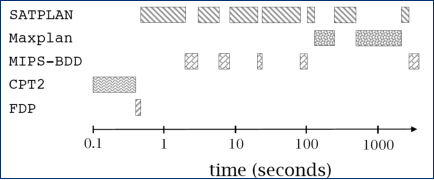•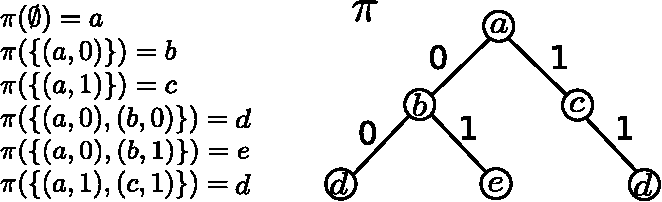Highly Cited
2007
Highly Cited
2007
• IPCO
• 2007
• Corpus ID: 10051765
Let $f:2^{N} \rightarrow \cal R^{+}$ be a non-decreasing submodular set function, and let $(N,\cal I)$ be a matroid. We consider… Expand
Highly Cited
2004
Highly Cited
2004
In this paper, we obtain an (1-e^-^1)-approximation algorithm for maximizing a nondecreasing submodular set function subject to a… Expand
Highly Cited
2001
Highly Cited
2001
• JACM
• 2001
• Corpus ID: 888513
This paper presents a combinatorial polynomial-time algorithm for minimizing submodular functions, answering an open question… Expand
•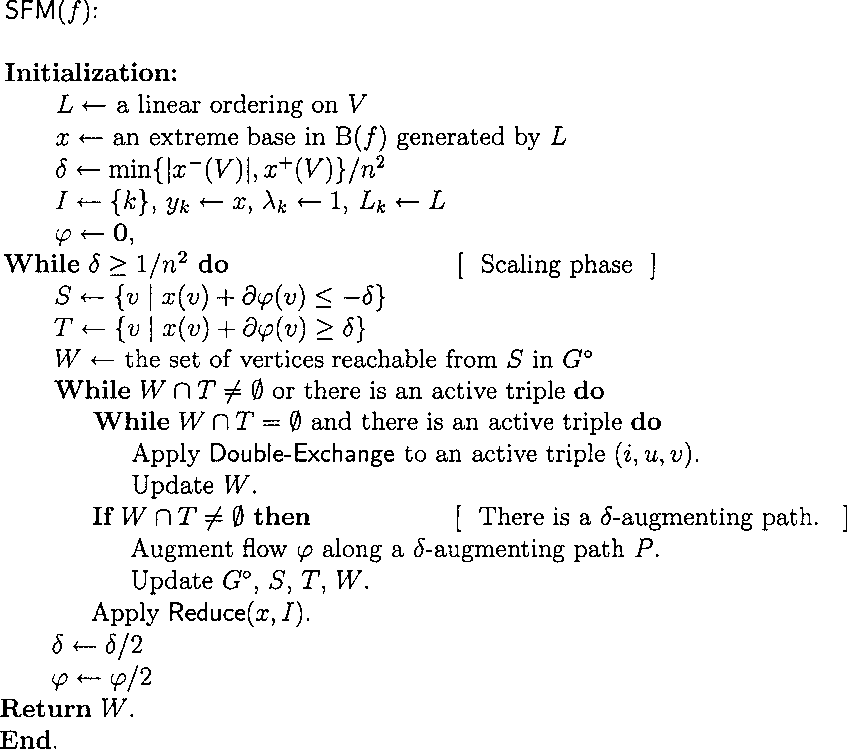•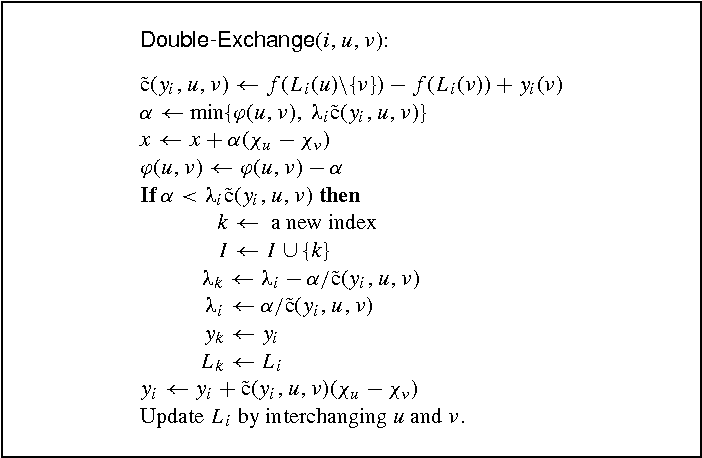•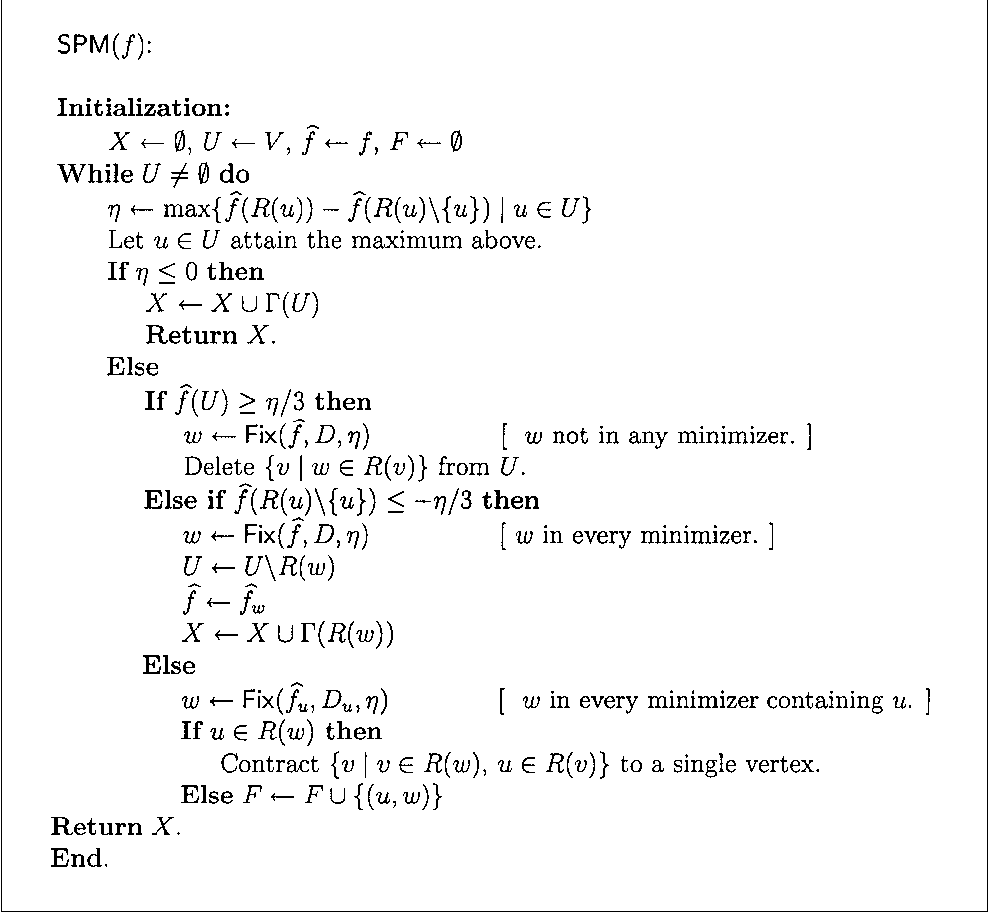Highly Cited
2000
Highly Cited
2000
We give a strongly polynomial-time algorithm minimizing a submodular function f given by a value-giving oracle. The algorithm… Expand
Highly Cited
1982
Highly Cited
1982
AbstractWe consider the problem: min $$\{ \mathop \Sigma \limits_{j \in s} f_j :z(S) = z(N),S \subseteqq N\}$$ wherez is a… Expand
Highly Cited
1978
Highly Cited
1978
• Math. Program.
• 1978
• Corpus ID: 206800425
LetN be a finite set andz be a real-valued function defined on the set of subsets ofN that satisfies z(S)+z(T)≥z(S⋃T)+z(S⋂T) for… Expand
Highly Cited
1978
Highly Cited
1978
This paper gives general conditions under which a collection of optimization problems, with the objective function and the… Expand
Highly Cited
1978
Highly Cited
1978
Given a finite set E and a real valued function f on P(E) (the power set of E) the optimal subset problem (P) is to find S ⊂ E… Expand
Highly Cited
1978
Highly Cited
1978
• Math. Oper. Res.
• 1978
• Corpus ID: 9027438
A real-valued function z whose domain is all of the subsets of N = {1,..., n is said to be submodular if zS + zT ≥ zS ∪ T + zS… Expand